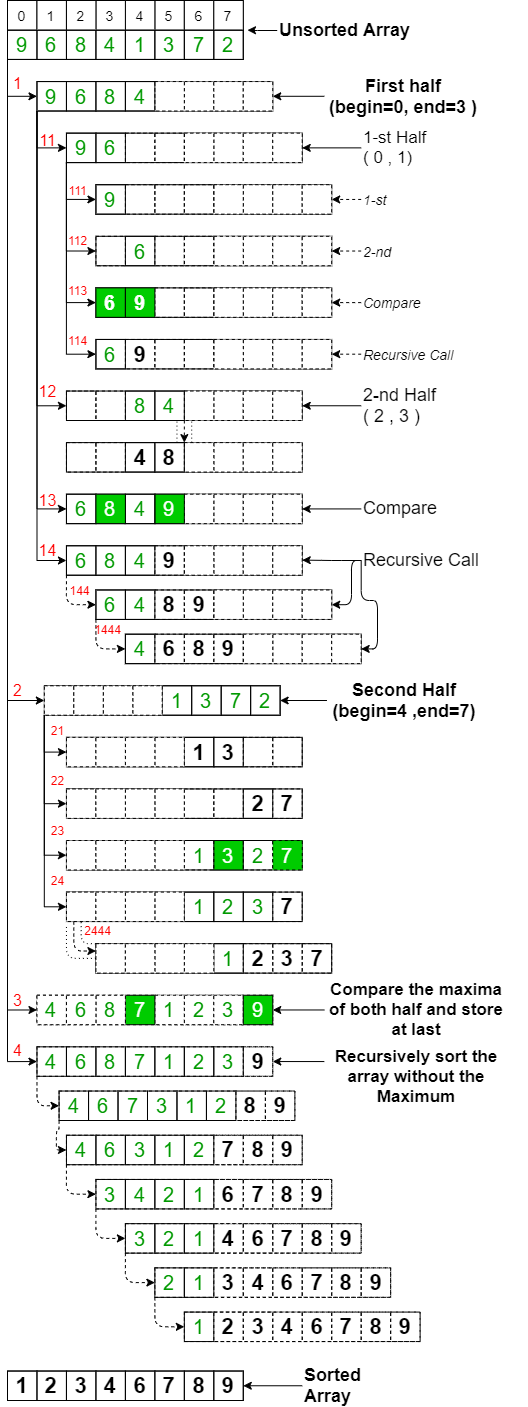Related Articles

# Sort the array using slow sort

• Last Updated : 22 Jun, 2021

Given an array arr[] consisting of N integers, the task is to sort the given array in ascending order using the slow sort.

Examples:

Input: arr[] = {6, 8, 9, 4, 12, 1}
Output: 1 4 6 8 9 12

Input: arr[] = {5, 4, 3, 2, 1}
Output: 1 2 3 4 5

Approach: Like Merge Sort, Slow Sort is a Divide and Conquer algorithm. It divides the input array into two halves, calls itself the two halves, and then compares the maximum element of the two halves. It stores the maximum element of a sub-array at the top position of the sub-array, then, it recursively calls the sub-array without the maximum element. Follow the steps below to solve the problem:

SlowSort(arr[], l, r):

• If r >= l, perform the following steps:
• Find the middle value of the array as m = (l + r) / 2.
• Recursively call function SlowSort to find the maximum of first half elements: SlowSort(arr, l, m)
• Recursively call function SlowSort to find the maximum of second-half elements: SlowSort(arr, m + 1, r)
• Store the largest of two maxima returned from the above function calls at the end as arr[r] = max(arr[m], arr[r])
• Recursively call function SlowSort without the maximum obtained in the above step: SlowSort(arr, l, r-1)

The following figure shows the complete Slow Sort process. For example, array {9, 6, 8, 4, 1, 3, 7, 2}. From the figure, it can be observed that the array is recursively divided into two halves till the size becomes 1. Once the size becomes 1, the comparison process begins.Slow Sort

Below is the implementation for the above approach:

## C++

 // C++ program for the above approach#include using namespace std; // Function to swap two elementsvoid swap(int* xp, int* yp){    int temp = *xp;    *xp = *yp;    *yp = temp;} // Function to sort the array using// the Slow sortvoid slow_sort(int A[], int i, int j){    // Recursion break condition    if (i >= j)        return;     // Store the middle value    int m = (i + j) / 2;     // Recursively call with the    // left half    slow_sort(A, i, m);     // Recursively call with the    // right half    slow_sort(A, m + 1, j);     // Swap if the first element is    // lower than second    if (A[j] < A[m]) {        swap(&A[j], &A[m]);    }     // Recursively call with the    // array excluding the maximum    // element    slow_sort(A, i, j - 1);} // Function to print the arrayvoid printArray(int arr[], int size){    int i;    for (i = 0; i < size; i++)        cout << arr[i] << " ";    cout << endl;} // Driver Codeint main(){    // Given Input    int arr[] = { 6, 8, 9, 4, 12, 1 };    int n = sizeof(arr) / sizeof(arr);     // Function Call    slow_sort(arr, 0, n - 1);     // Print the sorted array    printArray(arr, n);     return 0;}

## Java

 // Java program for the above approachclass GFG{ // Function to sort the array using// the Slow sortstatic void slow_sort(int A[], int i, int j){         // Recursion break condition    if (i >= j)        return;     // Store the middle value    int m = (i + j) / 2;     // Recursively call with the    // left half    slow_sort(A, i, m);     // Recursively call with the    // right half    slow_sort(A, m + 1, j);     // Swap if the first element is    // lower than second    if (A[j] < A[m])    {        int temp = A[j];        A[j] = A[m];        A[m] = temp;    }     // Recursively call with the    // array excluding the maximum    // element    slow_sort(A, i, j - 1);} // Function to print the arraystatic void printArray(int arr[], int size){    int i;    for(i = 0; i < size; i++)        System.out.print(arr[i] + " ");             System.out.println();} // Driver codepublic static void main(String[] args){    int arr[] = { 6, 8, 9, 4, 12, 1 };    int n = arr.length;     // Function Call    slow_sort(arr, 0, n - 1);     // Print the sorted array    printArray(arr, n);}} // This code is contributed by abhinavjain194

## Python3

 # Python3 program for the above approach # Function to sort the array using# the Slow sortdef slow_sort(A, i, j):         # Recursion break condition    if (i >= j):        return             # Store the middle value    m = (i + j) // 2         # Recursively call with the    # left half    slow_sort(A, i, m)     # Recursively call with the    # right half    slow_sort(A, m + 1, j)     # Swap if the first element is    # lower than second    if (A[j] < A[m]):        temp = A[m]        A[m] = A[j]        A[j] = temp     # Recursively call with the    # array excluding the maximum    # element    slow_sort(A, i, j - 1) # Function to print the arraydef printArray(arr, size):         for i in range(size):        print(arr[i], end = " ") # Driver Codeif __name__ == '__main__':         arr = [ 6, 8, 9, 4, 12, 1 ]    n = len(arr)         # Function Call    slow_sort(arr, 0, n - 1)         # Print the sorted array    printArray(arr, n) # This code is contributed by SoumikMondal

## C#

 // C# implementation of the approachusing System; class GFG{   // Function to sort the array using// the Slow sortstatic void slow_sort(int[] A, int i, int j){         // Recursion break condition    if (i >= j)        return;     // Store the middle value    int m = (i + j) / 2;     // Recursively call with the    // left half    slow_sort(A, i, m);     // Recursively call with the    // right half    slow_sort(A, m + 1, j);     // Swap if the first element is    // lower than second    if (A[j] < A[m])    {        int temp = A[j];        A[j] = A[m];        A[m] = temp;    }     // Recursively call with the    // array excluding the maximum    // element    slow_sort(A, i, j - 1);} // Function to print the arraystatic void printArray(int[] arr, int size){    int i;    for(i = 0; i < size; i++)        Console.Write(arr[i] + " ");             Console.WriteLine();}     // Driver code    public static void Main()    {    int[] arr = { 6, 8, 9, 4, 12, 1 };    int n = arr.Length;     // Function Call    slow_sort(arr, 0, n - 1);     // Print the sorted array    printArray(arr, n);    }} // this code is contributed by sanjoy_62.

## Javascript

 
Output:
1 4 6 8 9 12

Best Case Time Complexity:, where e > 0
Average Case Time Complexity:Auxiliary Space: O(1)

Attention reader! Don’t stop learning now. Get hold of all the important DSA concepts with the DSA Self Paced Course at a student-friendly price and become industry ready.  To complete your preparation from learning a language to DS Algo and many more,  please refer Complete Interview Preparation Course.

In case you wish to attend live classes with experts, please refer DSA Live Classes for Working Professionals and Competitive Programming Live for Students.

My Personal Notes arrow_drop_up